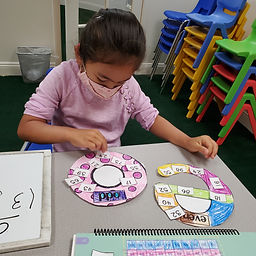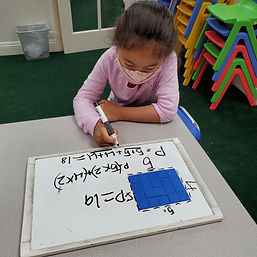## Ms. Raejean

### Target 1​

###### Lesson Type:

New

Number Operation

:

Number Classes

Understand that a whole number can be described as even or odd.

###### 1:

Find all the even and odd numbers on a hundred’s chart.

###### 2:

Understand that the digit in the ones’ place determines whether a number is even or odd.

###### 3:

Recognize that even numbers have a 0, 2, 4, 6, or 8 in the ones’ place.

###### 4:

Recognize that odd numbers have a 1, 3, 5, 7, or 9 in the ones’ place.

2nd

###### Vocabulary:

Odd, Even, Pattern, Place Value, Ones

Activities:

• Students shared what they know about odd and even numbers. Even numbers have a pair - 2, 4 , 6, 8, 10. Odd numbers don't have a partner - 1, 3, 5, 7, 9.
• Students used a hundreds chart to identify odd and even numbers. Even numbers were found by skip counting by 2.
• Students sorted 2-digit numbers as odd or even. Students referred to the hundreds chart as needed.
• Students were asked how they know a number is odd or even. They looked at the ones place. Even numbers have 2, 4, 6, 8, 0 in the ones place, while odd numbers have 1, 3, 5, 7, 9 in the ones place.### Home Exploration

###### Guiding Questions:## Absent Students:

### Target 2

:

###### 1:

Identify the pattern that occurs when two even numbers are added.

###### 2:

Identify the pattern that occurs when two odd numbers are added.

###### 3:

Identify the pattern that occurs when an even and odd number are added.

3rd

###### Vocabulary:

Odd, Even, Pattern, Place Value, Ones, Sum

Activities:

• Students identified each number in addition equations as odd or even. Then students found the sum and identified it as odd or even. Finally, students noticed the patterns. Even + Even = Even, Odd + Odd = Even, Even + Odd = Odd### Home Exploration

###### Guiding Questions:### Target 3

:

###### 1:

Define perimeter.

###### 2:

Understand that perimeter is the distance around a figure.

###### 3:

Understand that perimeter can be found by adding the lengths of the sides of a figure.

3rd

###### Vocabulary:

Perimeter

Activities:

• We reviewed perimeter - the edges around a shape. Students used pentominoes to build various shapes and found the perimeter.
• Students are working on writing equations to find the perimeter by adding each side of the shape. Side + Side + Side + Side = 5 + 5 + 3 + 3### Home Exploration